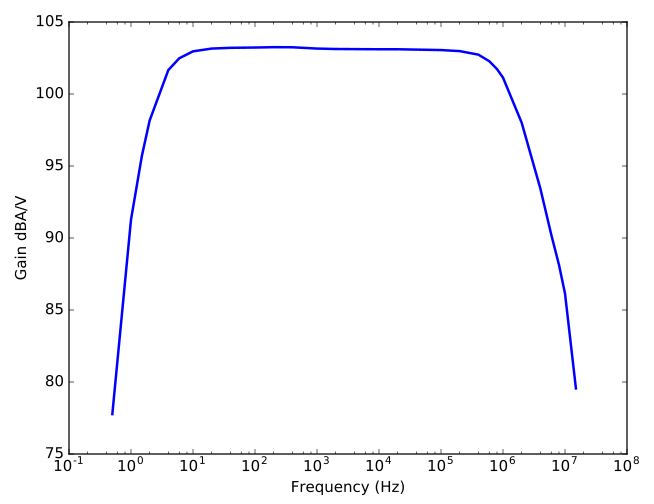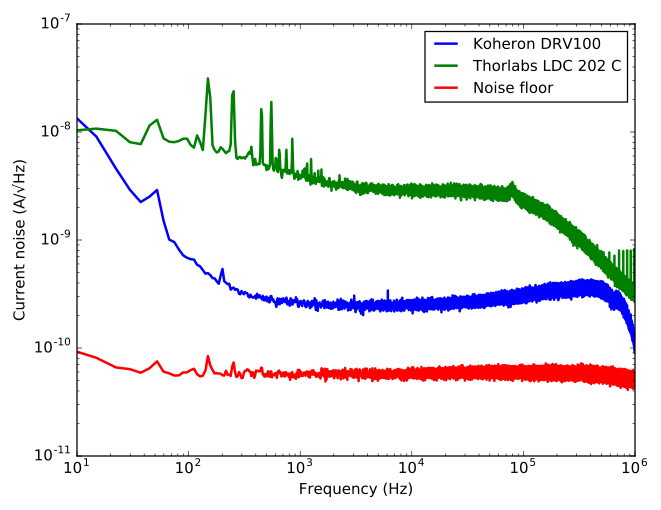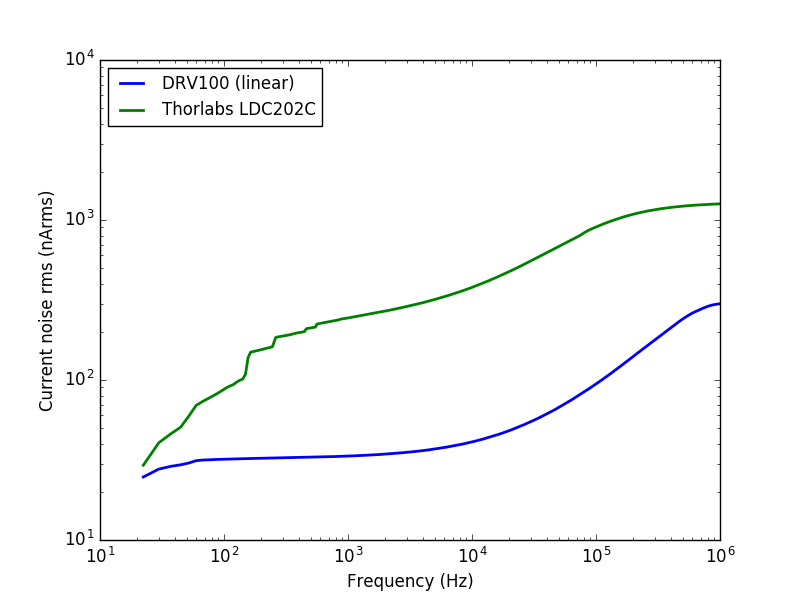# Measuring the noise of laser current sources

2017-03-24

Laser diodes are very high efficiency devices: a photon is emitted for almost each injected electron. As a consequence, emitted light closely mimics the behavior of the driving current source. A low noise current driver is required to reach high quality emission. But what does "low noise" mean?

## Power spectral density

A standard definition often used in electronics is the peak-peak amplitude in a 10 Hz to 100 kHz bandwidth. Such a definition provides a single value that can be compared across products, but since it is an integrated value, it does not provide any information on how the noise is distributed across the frequencies.

To get a precise knowledge of the noise, the power spectral density is used. The power spectral density S(f) at frequency f is defined as the power passing through a band-pass filter of width 1 Hz centered at f. The unit of S is W/Hz.

For example, the power spectral density associated with the thermal noise in a resistor at temperature T is $S = 4 k_{B} T$ where kB is the Boltzmann constant. Note that it is independent of the resistor value. It is also independent of the frequency f so the thermal noise is a white noise. Since the power dissipated by a resistor R is $P = R I^{2}$, the resistor current noise density is:

$$i_{n} = \sqrt{\frac{S}{R}} = 2 \sqrt{\frac{k_{B} T}{R}},$$

whose unit is A/√Hz. For a 1 kΩ resistor at a temperature of 300 K, the current noise density is 4 pA/√Hz. The related rms noise in a 10 Hz to 100 kHz bandwidth is:

$$I_{\rm rms} = \sqrt{ \int_{\rm 10 Hz}^{\rm 100 kHz} i_{n}^{2}(f) \, df } = i_{n} \sqrt{\rm 100 kHz - 10 Hz}.$$

For a 1 kΩ resistor, this gives 1.3 nArms.

## Measuring the current noise density

In this setup, we will use the Koheron DRV100, a low noise laser diode driver, to measure its current noise density.

For a low noise current driver, current fluctuations can be more than 100 000 times smaller than the average current.

Here is an overview of a setup dedicated to observe such tiny ripples:The current provided by Koheron DRV100 is converted into a voltage through a high-quality precision resistor Rs. The resulting voltage is then AC coupled using a pair of capacitors before being amplified with an instrumentation amplifier of gain G. The figure below shows the frequency response of our amplifier:The 3 dB bandpass response is from 2.8 Hz to 1.3 MHz. The amplifier gain is 145.4 kV/A. The signal at the amplifier output is acquired using an analog-to-digital converter. The digitized signal is then down-sampled on a FPGA.

Following , we can compute the power spectral density from the acquired time series. Let N be the number of points in the time series and xk the samples of the time series (converted in Volts from ADC calibration). The discrete Fourier transform is:

$$y_{m} = \sum_{k=0}^{N-1} w_{k} x_{k} e^{- 2 \pi i mk / N},$$

where wk is a chosen window. The power spectral density is:

$$S_{m} = \frac{2}{f_{s} W} |y_{m}|^{2},$$

where fs is the sampling frequency and $W = \sum_{k=0}^{N-1} w_{k}^{2}$. Taking the square root of the power spectral density and scaling by the amplifier gain, we obtain the current noise density in A/√Hz.

## Results

We measured the current noise density between 10 Hz and 1 MHz for two current drivers: the Koheron DRV100 and the Thorlabs® LDC 202 C driver:The noise floor of the sensing amplifier is also depicted and it reaches 60 pA/√Hz.

By integrating the measured spectral densities, we obtain the rms noise amplitude versus the frequency bandwidth:: G. Heinzel, A. Rüdiger, and R. Schilling. Spectrum and spectral density estimation by the Discrete Fourier transform (DFT), including a comprehensive list of window functions and some new at-top windows. 2002